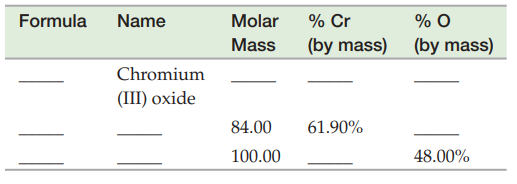Introductory Chemistry - 5 Edition - Chapter 6 - Problem 118p
Register Now

Join StudySoup

Get Full Access to Introductory Chemistry - 5 Edition - Chapter 6 - Problem 118p9780321910295

# Complete the table of compounds that contain only chromium and oxygen.FormulaNameMolar

Introductory Chemistry | 5th Edition

Problem 118P

Complete the table of compounds that contain only chromium and oxygen.Accepted Solution
Step-by-Step Solution:
Step 1 of 3

Solution 118P

The number of atoms in 1 mole of atoms is given by the avogadro's number named after amedeo avogadro its value is given by

1 mol = 6.023*1023.

The numerical value of mole is defined being equal to number of atoms in exactly 12 g of pure carbon .

###### Chapter 6, Problem 118P is Solved

Step 2 of 3

Step 3 of 3

Unlock Textbook Solution